# Top 35 Number Puzzles to Keep You Challenged

A logic number puzzle or triangle number puzzle might be a great resource to make learning math subjects enjoyable. Additionally, number puzzles develop strategic thinking, numeracy, and problem-solving skills.If you enjoy solving puzzles and are looking for some number puzzles to challenge your mind, you are in the right place. Through this blog, we will be sharing the best number puzzles for both adults and kids.

Plus, by exercising with these number puzzles you can also learn how to solve missing number puzzles and much more. Let’s get started!

## The Best Number Puzzle Games

It is clear that solving number puzzles is critical to practicing cognitive and math skills. Still, not everyone may enjoy them. So if you are thinking about how to solve number puzzles in a more fun way, the answer is hidden in gamification.

Here are the best puzzle games that can make solving puzzles enjoyable for both you and your kids: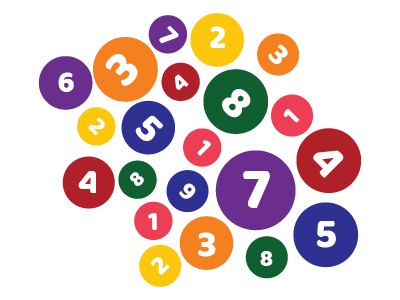Logic Number Puzzle

This entertaining puzzle will boost your math and visual attention skills at the same time.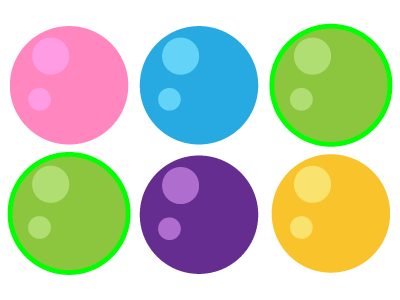Fun Number Puzzle

If you like visual number puzzles, you can solve them to improve your counting skills on short notice.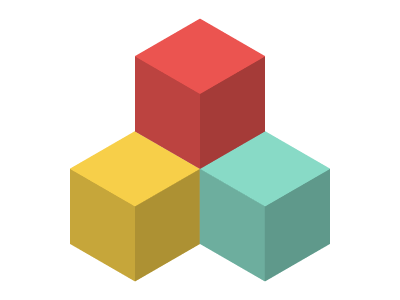Tricky Number Puzzle

When you want to develop your spatial perception skills with your kids, you can solve this puzzle.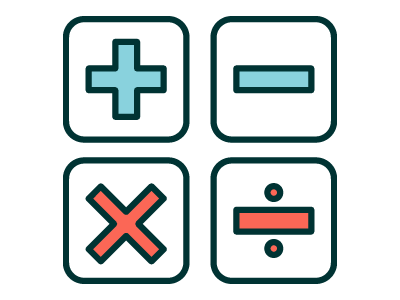Cross Number Puzzle

You can empower both your and your kids’ processing speed skills by having fun with this puzzle.

When the task is to complete a puzzle, turning it into a game enables individuals to focus better, concentrate longer, and enjoy the experience more. Therefore, number puzzle games are essential to having a good time while enhancing cognitive skills.

If you are looking for more puzzles to enjoy with your family members and support your kid's mental development, you can check out the puzzles within the MentalUP app. 👪

From logic puzzles to math puzzles, number puzzles to visual puzzles, all the puzzles that will benefit your kid's attention, logical thinking, problem-solving, and overall learning ability are here! 🚀

It is time to use the app right now to expand your kid's potential anytime, anywhere with only 20 minutes of daily MentalUP time! ✨

## The Most Entertaining Number Puzzles for Kids

If you find it hard to convince your kid to study math subjects and wonder what are some number puzzles will spark their interest in math and help them think and solve math problems faster, you are in luck!

Here are the most enjoyable number puzzles for kids!

1

### Logic Number Puzzle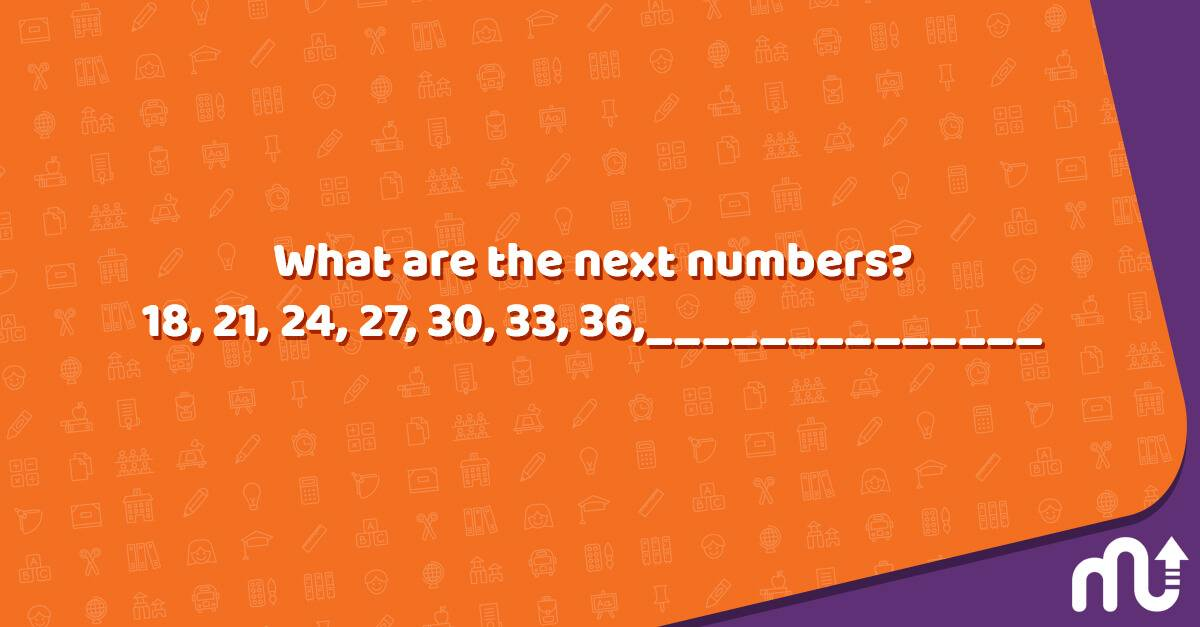Question: What are the next numbers? 18, 21, 24, 27, 30, 33, 36, ______________

Answer: Every successive number is greater by 3. Therefore, the sequence will be followed as 39, 42, 45, and so on.
2

### Age Puzzle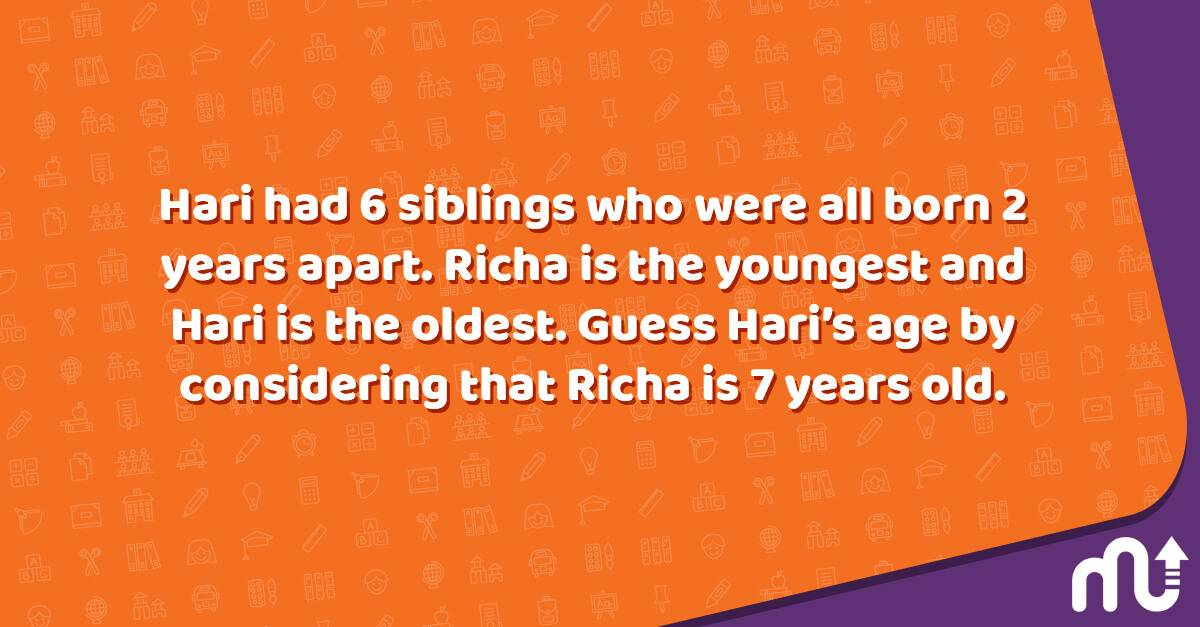Question: Hari had 6 siblings who were all born 2 years apart. Richa is the youngest and Hari is the oldest. Guess Hari’s age by considering that Richa is 7 years old.

Answer: Each sibling was born 2 years apart, and there are a total of seven children (Hari and his 6 siblings). So Hari’s age is: 7 + 2 + 2 + 2 + 2 + 2 + 2 = 19.
3

### A Tricky Number Puzzle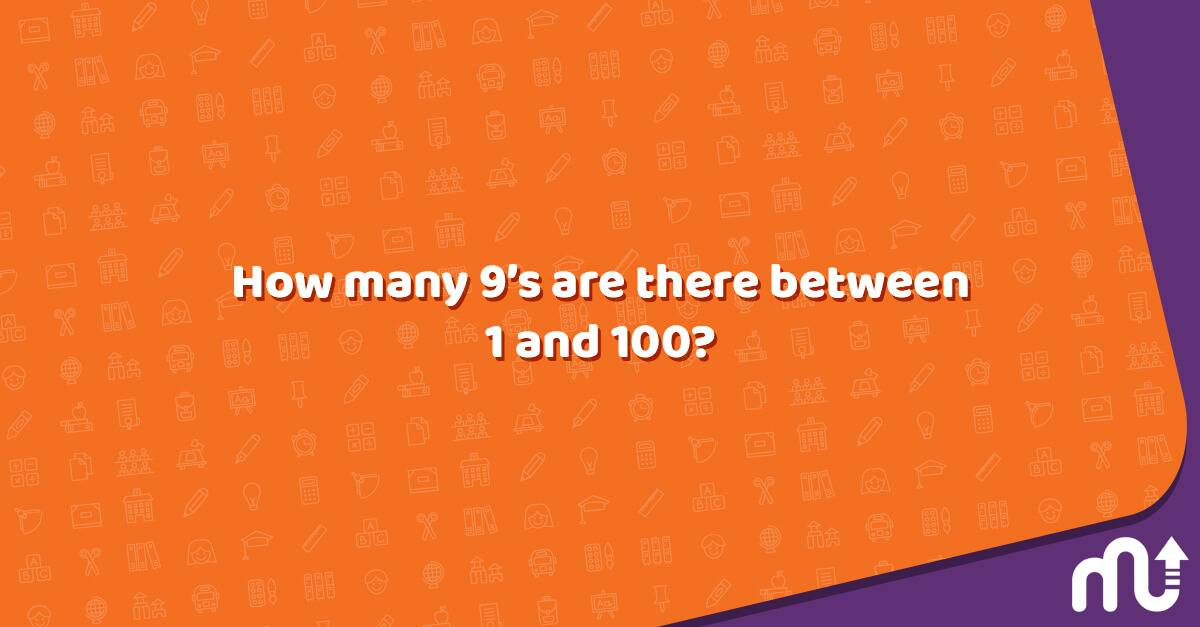Question: How many 9’s are there between 1 and 100?

Answer: 20. They are 9,19,29,39,49,59,69,79,89,90,91,92,93,94,95,96,97,98, and 99.
4

### Upside Down Puzzle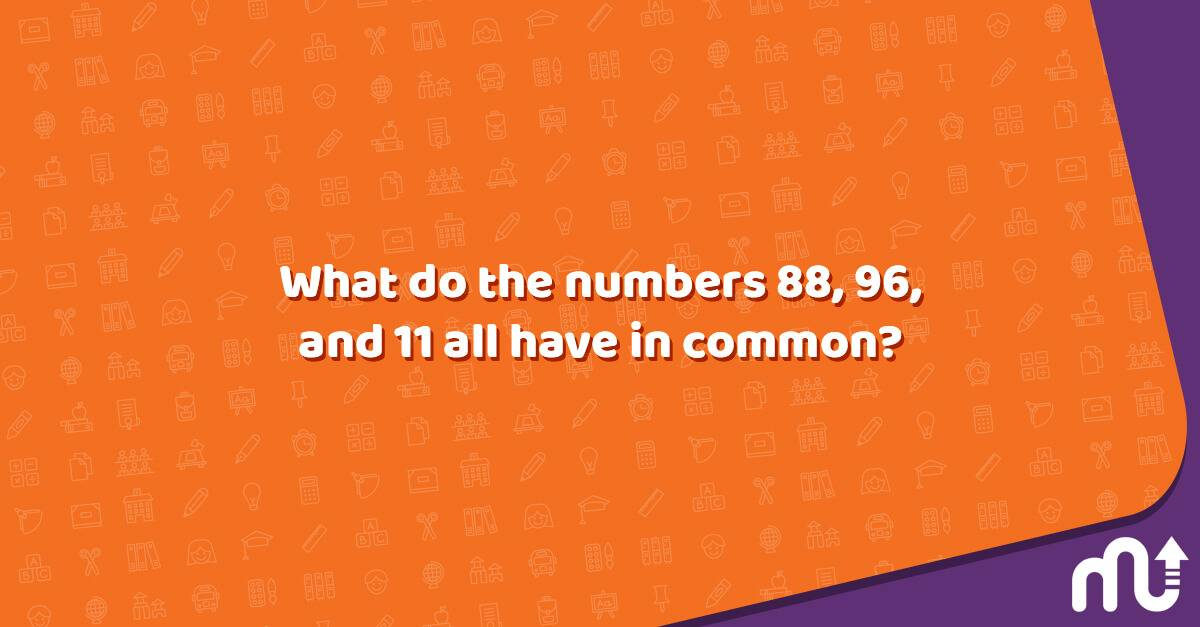Question: What do the numbers 88, 96, and 11 all have in common?

Answer: They look the same upside down and right side up.
5

### Number Grid Puzzle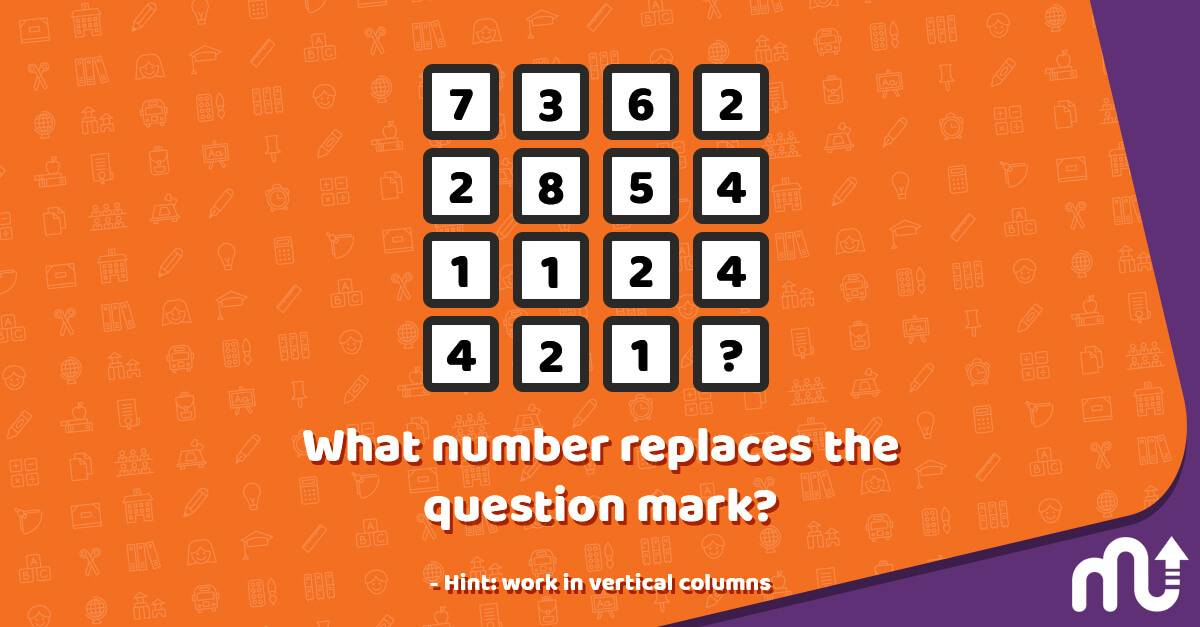Question: What number replaces the question mark? - Hint: work in vertical columns

Answer: The sum of the numbers in each column is always 14. So, the answer is 4.
6

### Riddle Puzzle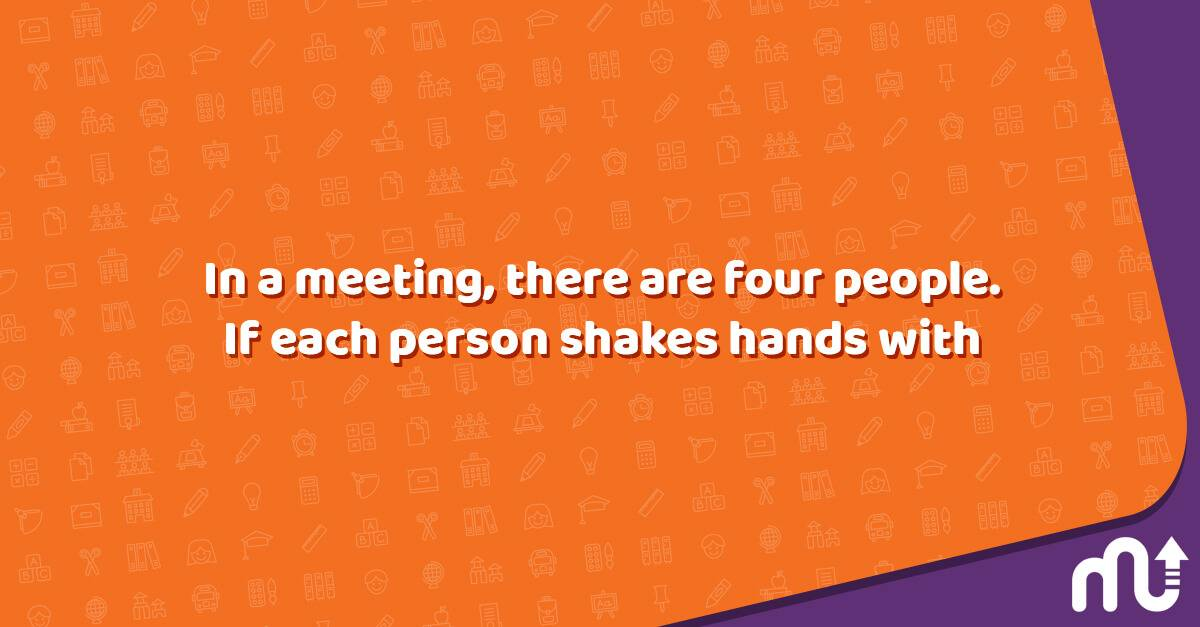Question: In a meeting, there are four people. If each person shakes hands with the other, how many handshakes will happen?

Answer: Make a list of all the handshakes made by each person. There will be three handshakes per person; however, the handshakes made by A to B and B to A are the same. So, after removing such repetitions, the answer is 6 handshakes.
7

### A Challenging Number Puzzle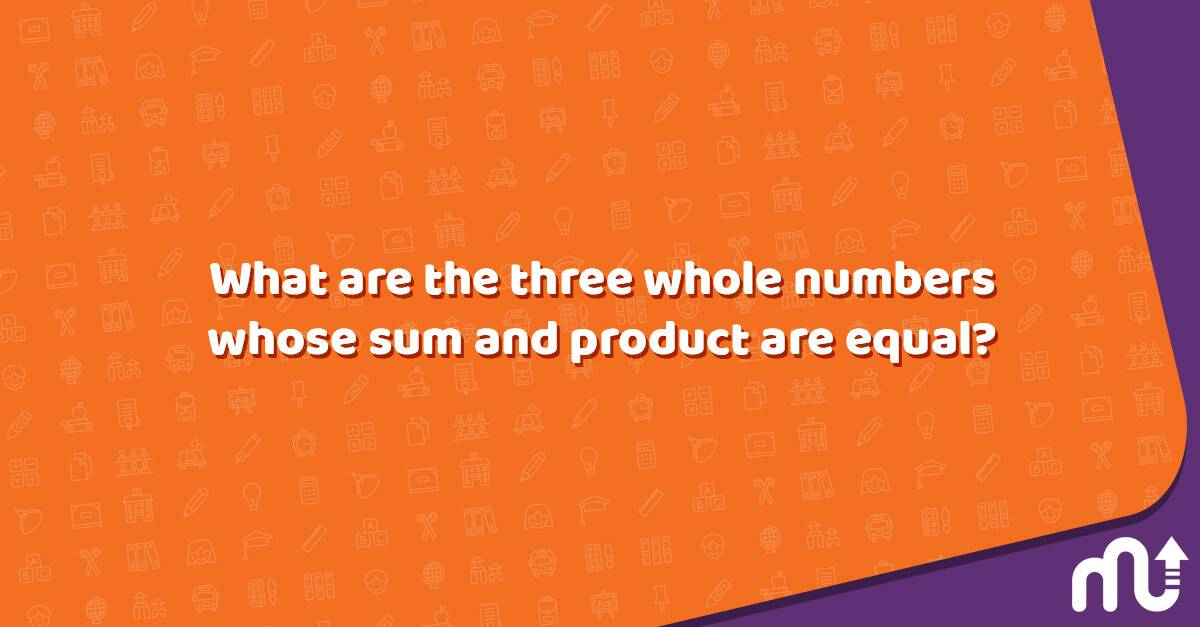Question: What are the three whole numbers whose sum and product are equal?

Answer: 1*2*3 = 6 and 1+2+3= 6. So, the numbers are 1,2, and 6.
8

### Fill-In Number Puzzle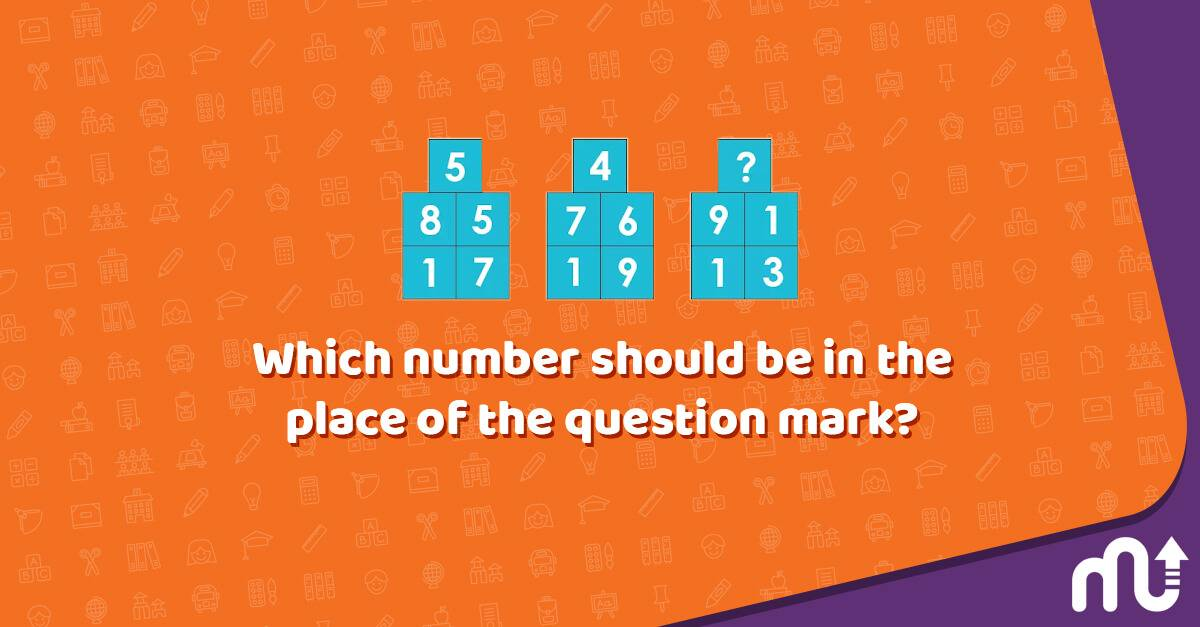Question: Which number should be in the place of the question mark?

Answer: The sequence of numbers is 85/17=5, 76/19=4, and 91/13=7. So, the answer is 7.
9

### Multiplication Puzzle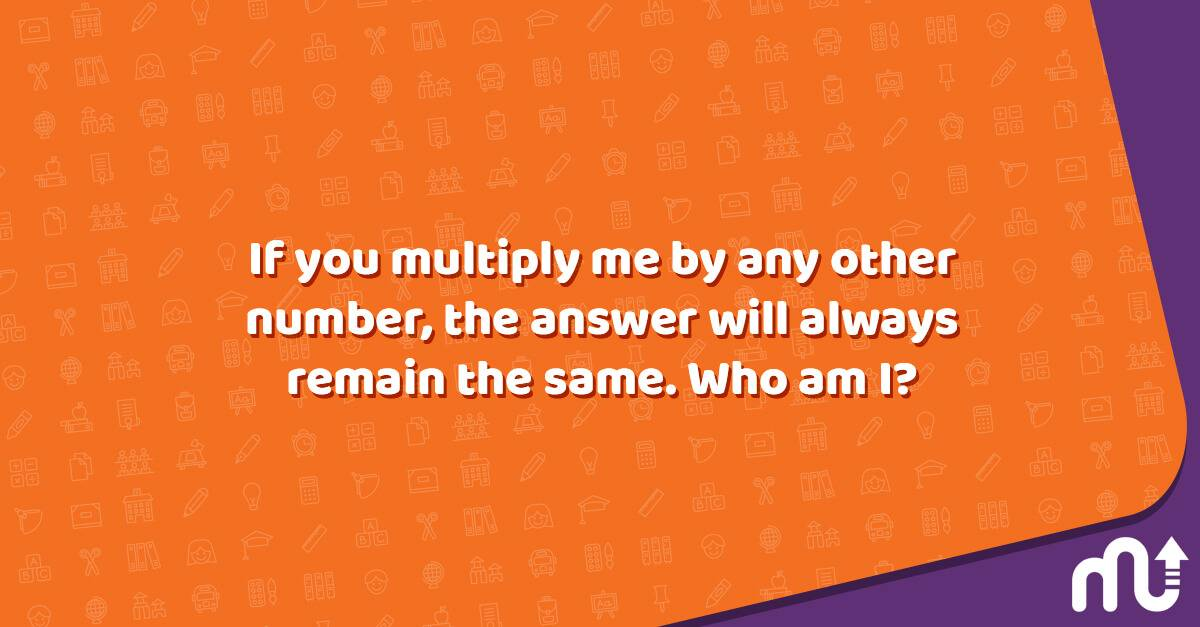Question: If you multiply me by any other number, the answer will always remain the same. Who am I?

10

### Price Puzzle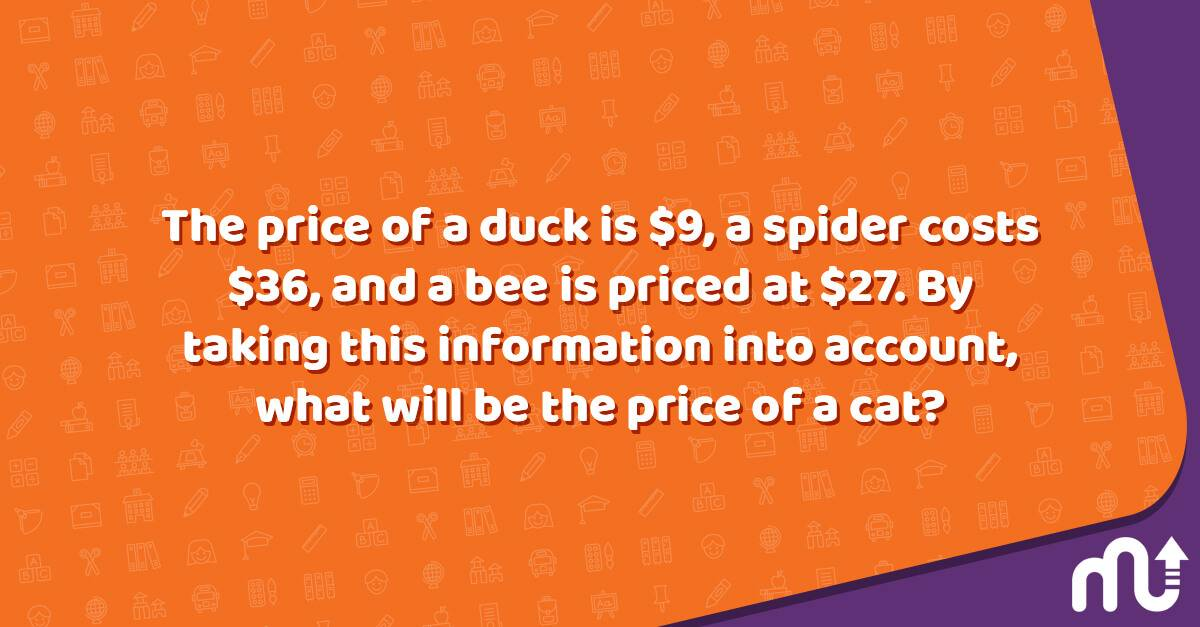Question: The price of a duck is \$9, a spider costs \$36, and a bee is priced at \$27. By taking this information into account, what will be the price of a cat?

11

### Odd Number Puzzle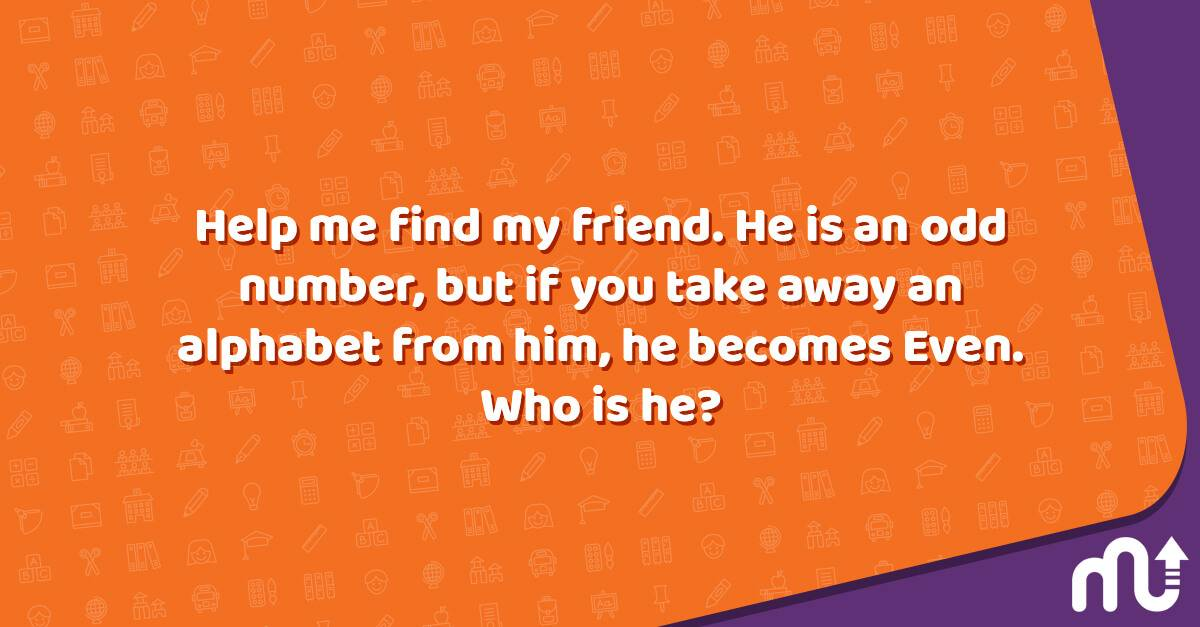Question: Help me find my friend. He is an odd number, but if you take away an alphabet from him, he becomes Even. Who is he?

Answer: When the letter “s” is taken out, the seven becomes even. So, the number is 7.
12

### Tricky Subtraction Puzzle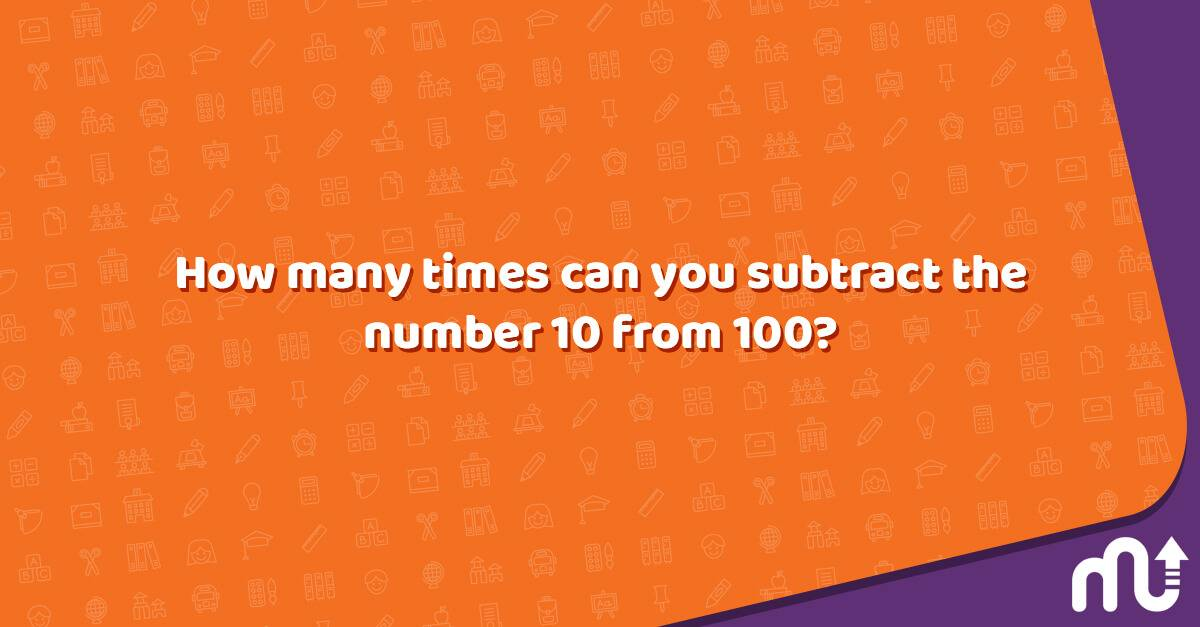Question: How many times can you subtract the number 10 from 100?

Answer: One time, because when you subtract, it is no longer 100.
13

### Multiplying Puzzle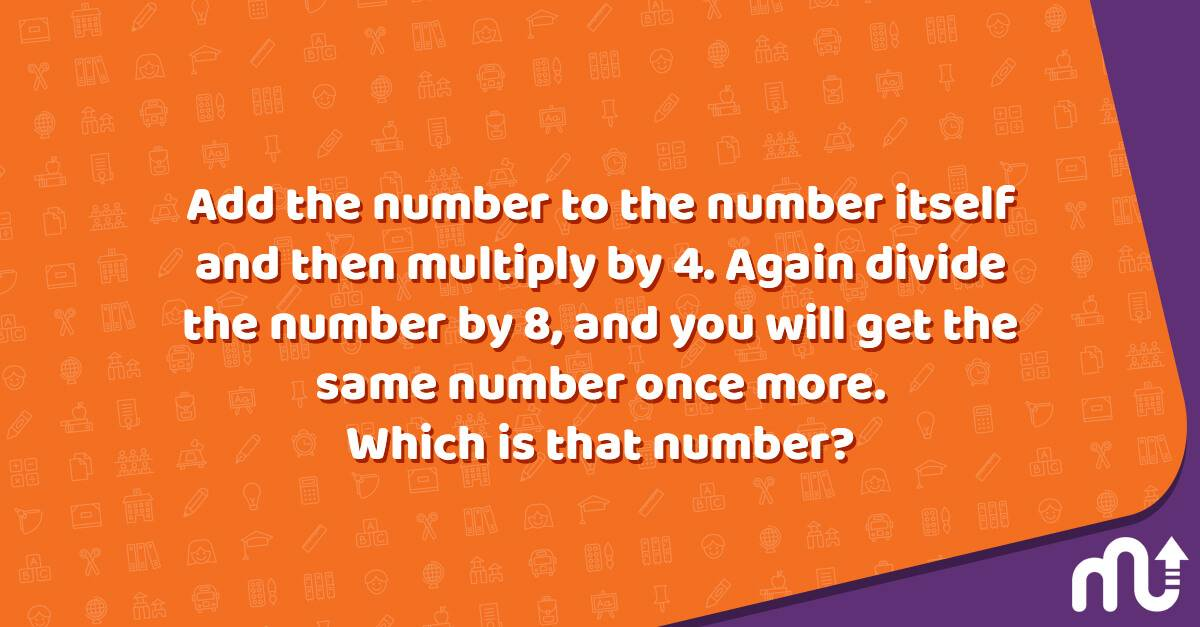Question: Add the number to the number itself and then multiply by 4. Again divide the number by 8, and you will get the same number once more. Which is that number?

Answer: Any number. For example, choose the number 2: 2+2=4, 4x4=16; then divide the number by 8, and you will get 2.
14

### Fruits Puzzle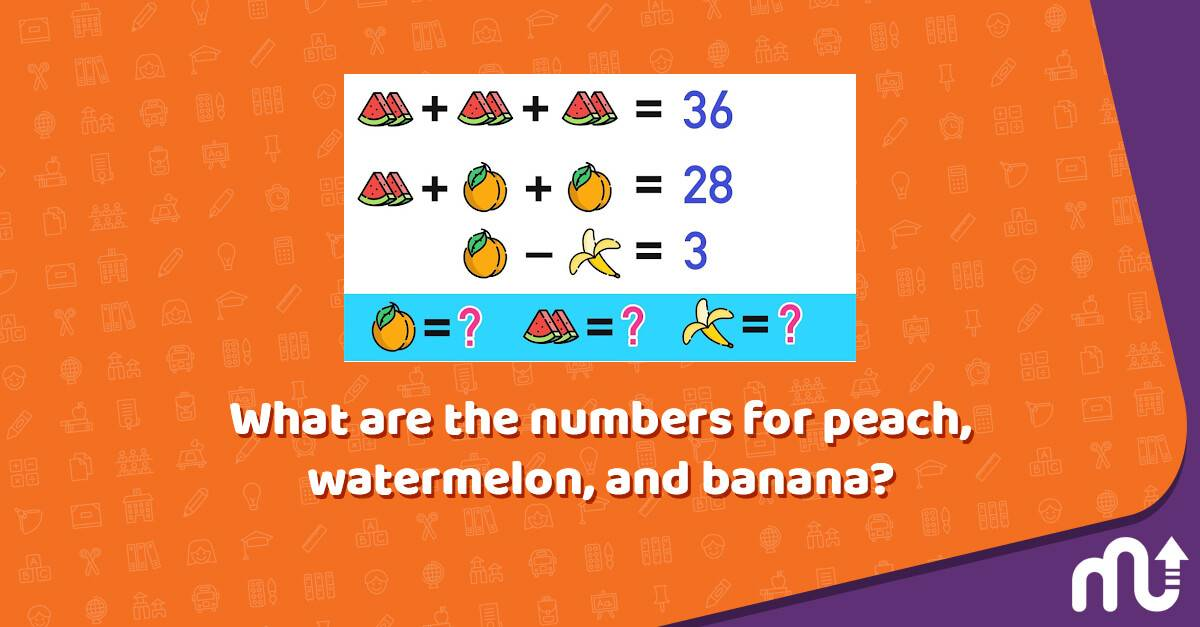Question: What are the numbers for peach, watermelon, and banana?

Answer: Peach= 8, Watermelon= 12, and Banana= 5
15

### Missing Numbers Puzzle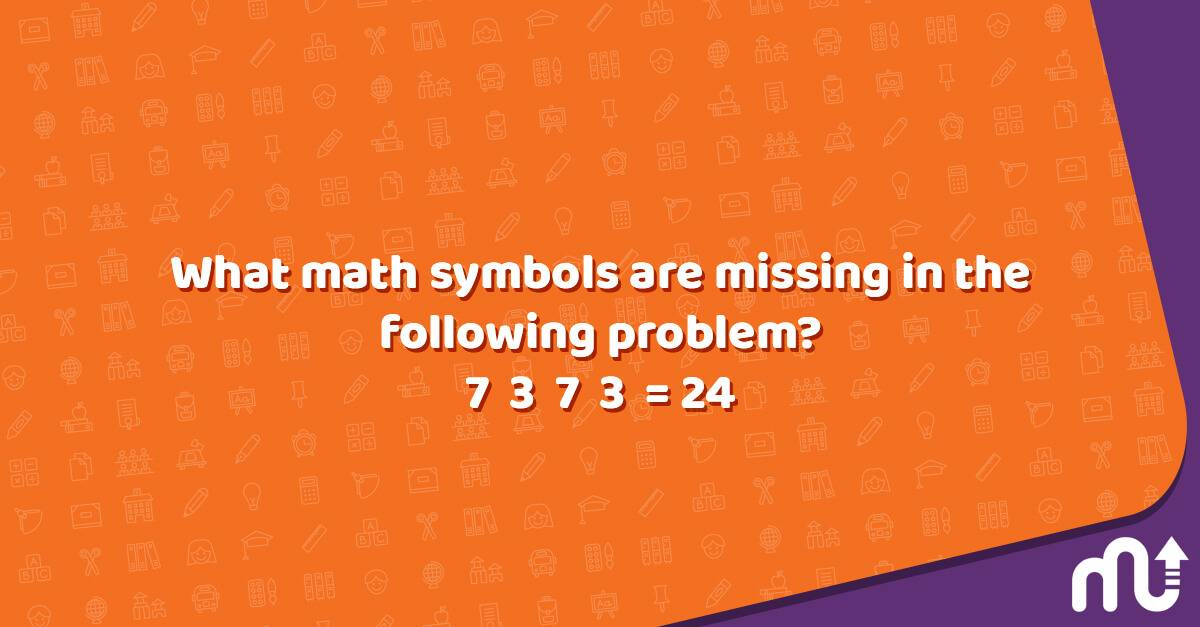Question: What math symbols are missing in the following problem? 7 3 7 3 = 24

Answer: 7 x (3/7 + 3)= 24
16

### Ball Puzzle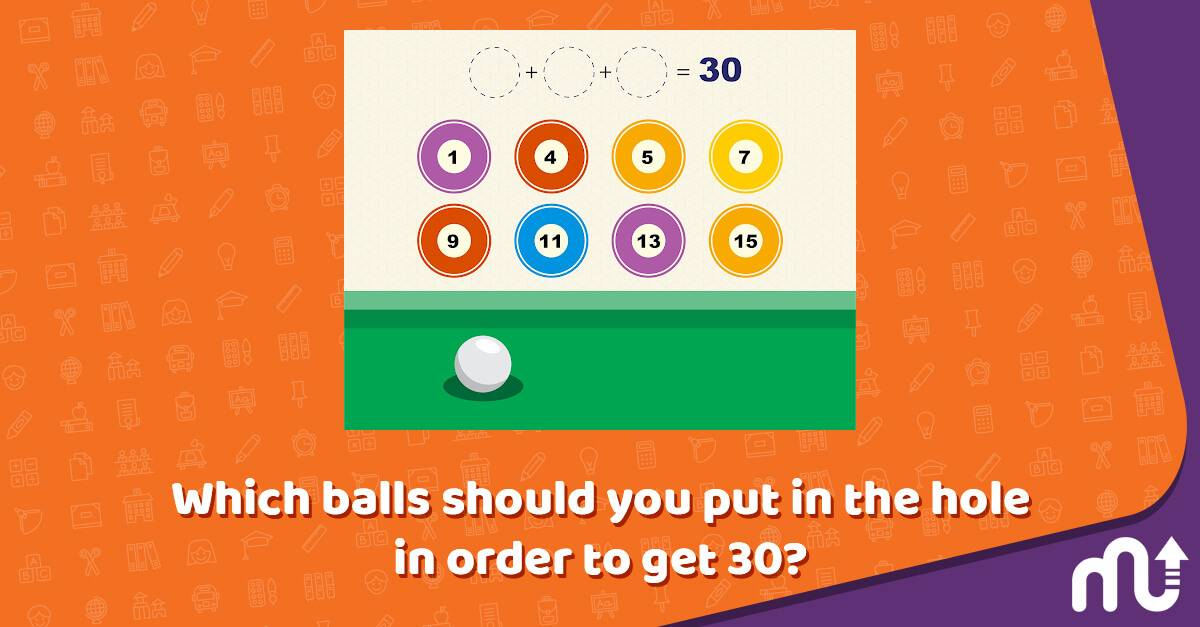Question: Which balls should you put in the hole in order to get 30?

17

### Chart Puzzle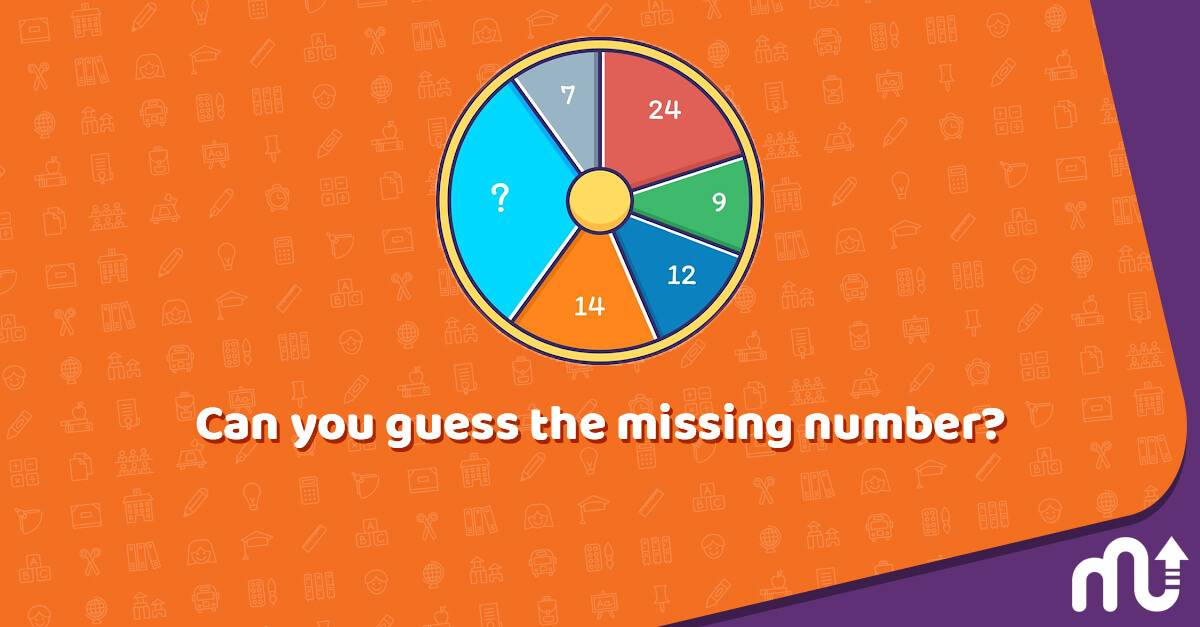Question: Can you guess the missing number?

Answer: The wheel is a pie chart. Each number and section represents the percentage. So 100 – 66 (total of the numbers) = 34.
18

### Weight Puzzle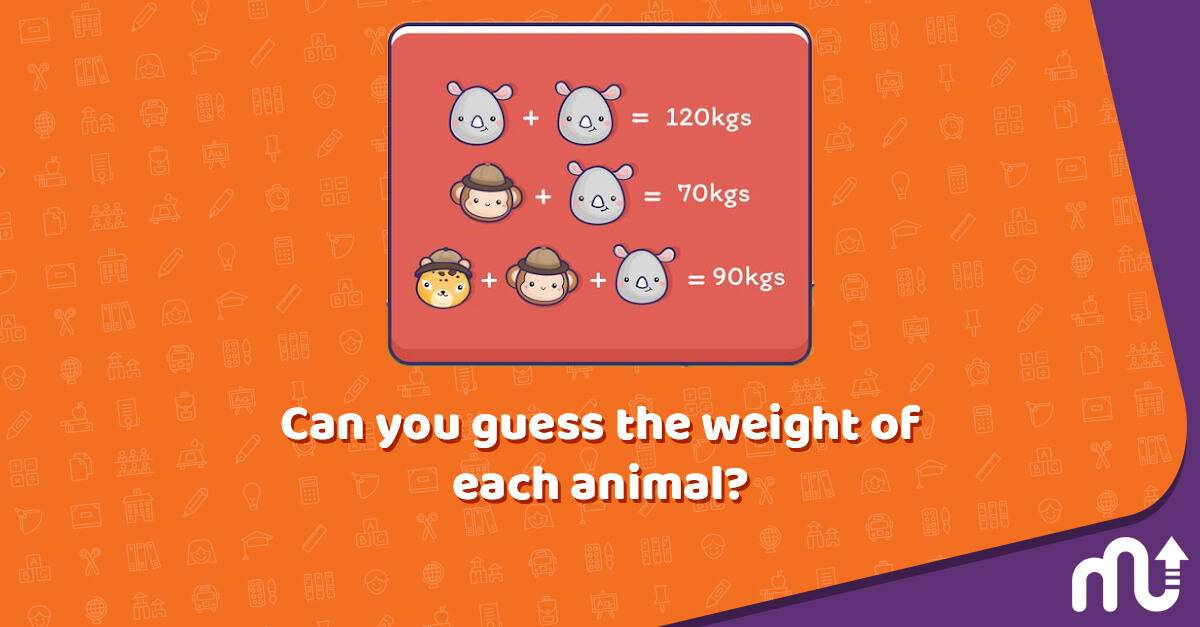Question: Can you guess the weight of each animal?

Answer: The rhino is 60, the monkey is 10, and the lion is 20 kilos.
19

### Tricky Logic Puzzle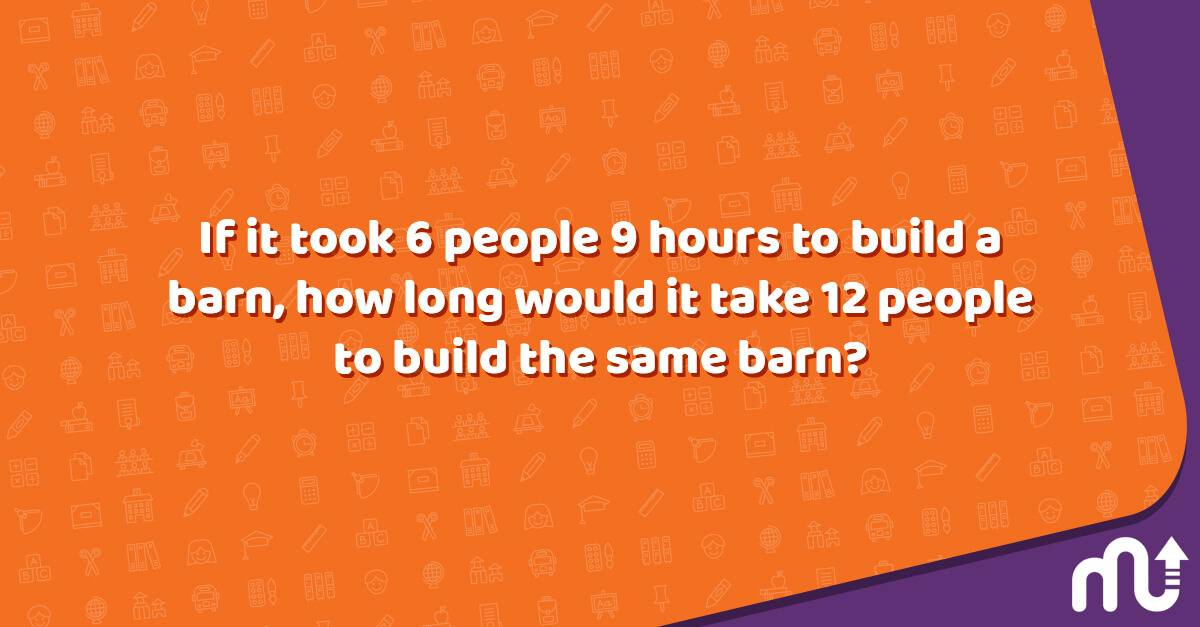Question: If it took 6 people 9 hours to build a barn, how long would it take 12 people to build the same barn?

20

### Hour Puzzle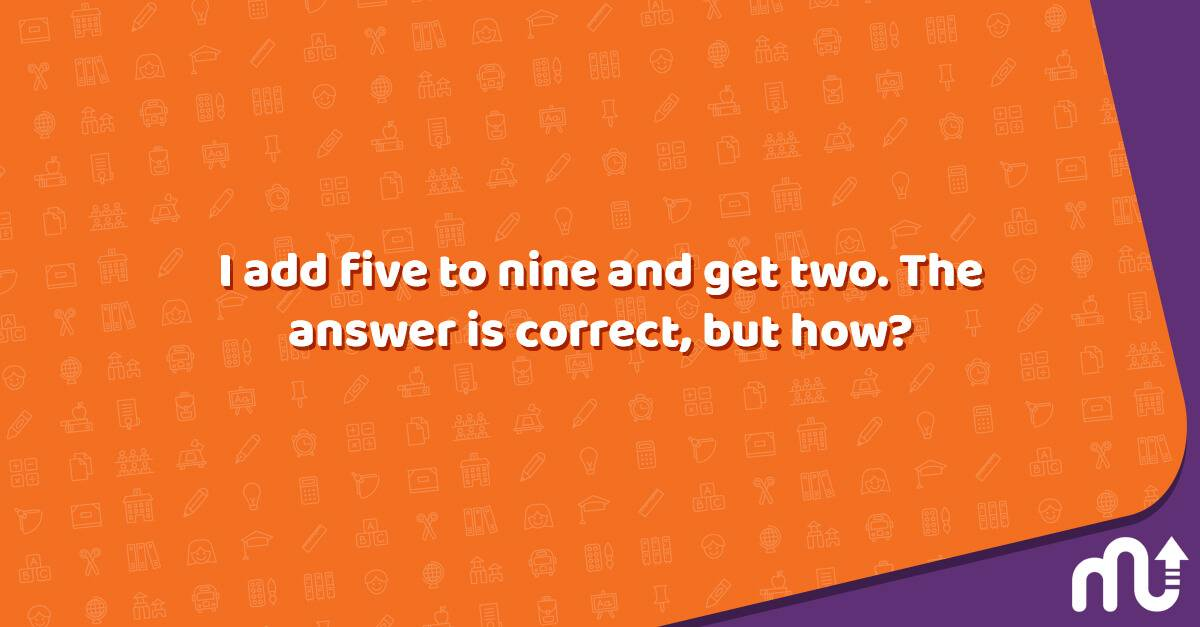Question: I add five to nine and get two. The answer is correct, but how?

Answer: When it is 9 AM, add 5 hours to it, and you will get 2 PM.

These number puzzles are great for sparking kids' cognitive skills and helping them practice learning how to solve missing number puzzles, grid puzzles, or logic puzzles. But practicing with the same puzzles can be boring for your kids.

You can utilize the MentalUP app to reach 150+ brain games and activities to accelerate your kid's mental development. 🌈

MentalUP provides your kid with a safe learning environment in which they can improve their creativity, attention, logical thinking, problem-solving, and analytical thinking skills with a variety of fun and entertaining activities. 🌟

You can benefit from MentalUP right now to reach age-appropriate memory, concentration, visual intelligence, logic, and fitness games to support your kid's mental and physical development! 🎉

## Popular & Fun Number Puzzles for Adults

So far, we have discovered the most fun and beneficial number puzzles for kids, but adults also need to exercise their minds with some entertaining number puzzles.

Let’s discover the most popular puzzles for adults to keep your mind challenged and trigger your cognitive skills.

1

### Digits Puzzle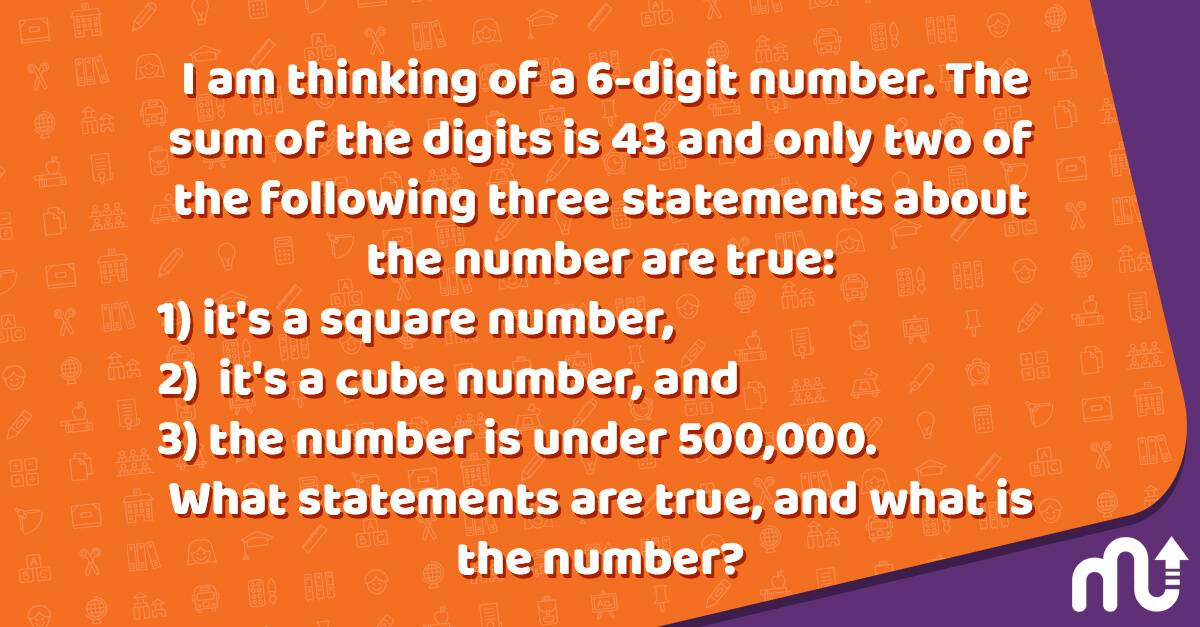Question: I am thinking of a 6-digit number. The sum of the digits is 43 and only two of the following three statements about the number are true: 1) it's a square number, 2) it's a cube number, and 3) the number is under 500,000. What statements are true, and what is the number?

Answer: Only statements 1 and 3 are true. The number is 499,849.
7,072 = 499,849
499,849 < 500,000
2

### Triangle Puzzle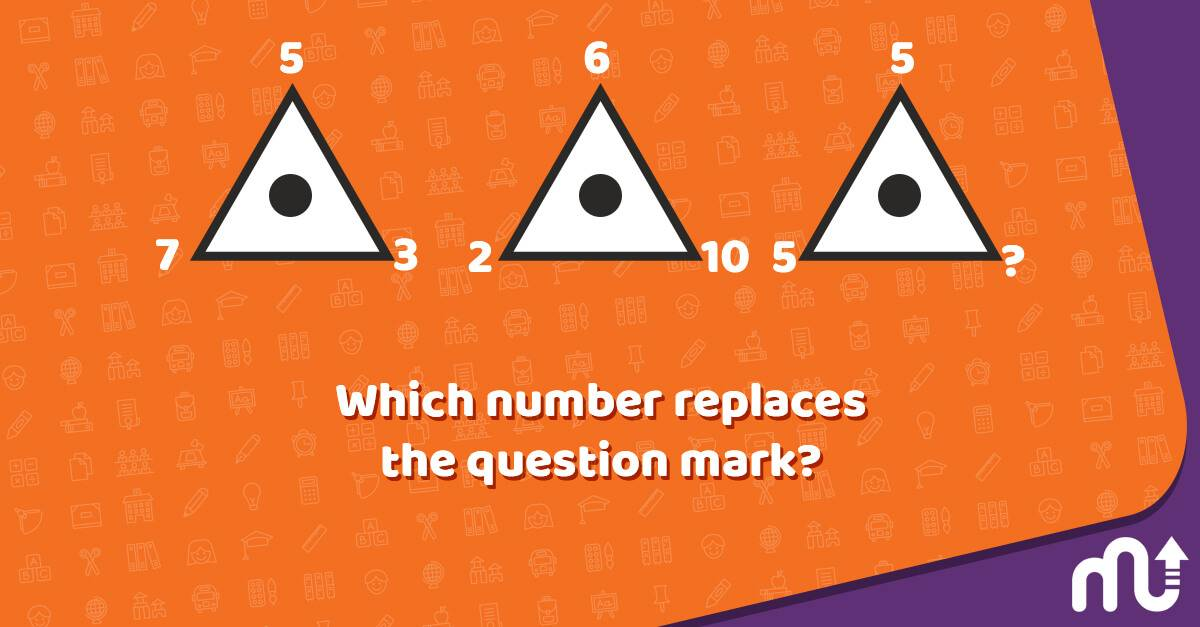Question: Which number replaces the question mark?

Answer: In each triangle, the number at the apex of the triangle equals the average of the two numbers at the bottom. So, the answer is 5.
3

### A Challenging Grid Puzzle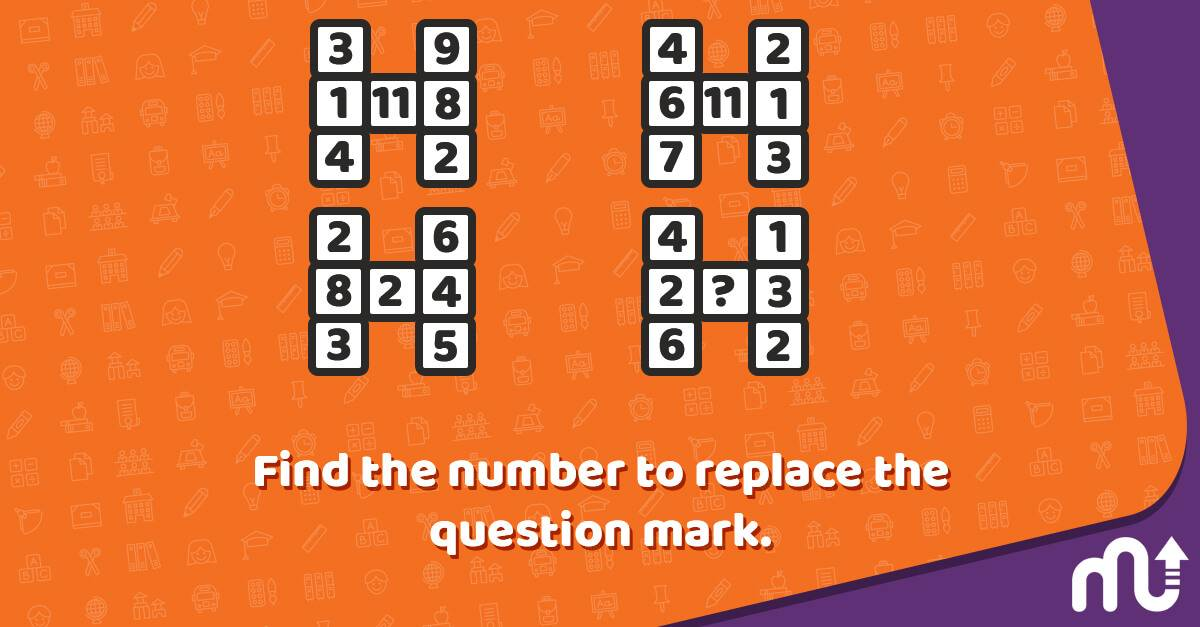Question: Find the number to replace the question mark.

Answer: In each diagram, the central value equals the difference between the sums of the numbers in the left-hand column and the sums of the numbers in the right-hand column. So, the answer is 6.
4

### Number 8 Puzzle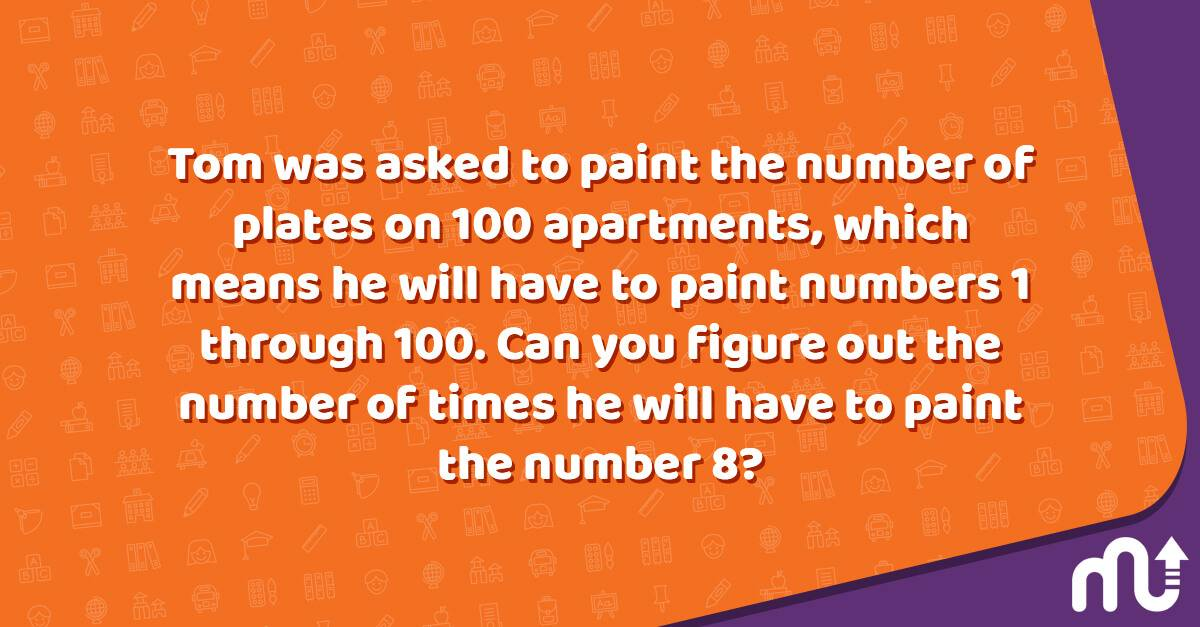Question: Tom was asked to paint the number of plates on 100 apartments, which means he will have to paint numbers 1 through 100. Can you figure out the number of times he will have to paint the number 8?

Answer: 20 times. (8, 18, 28, 38, 48, 58, 68, 78, 80, 81, 82, 83, 84, 85, 86, 87, 88, 89, 98)
5

### Sequence Puzzle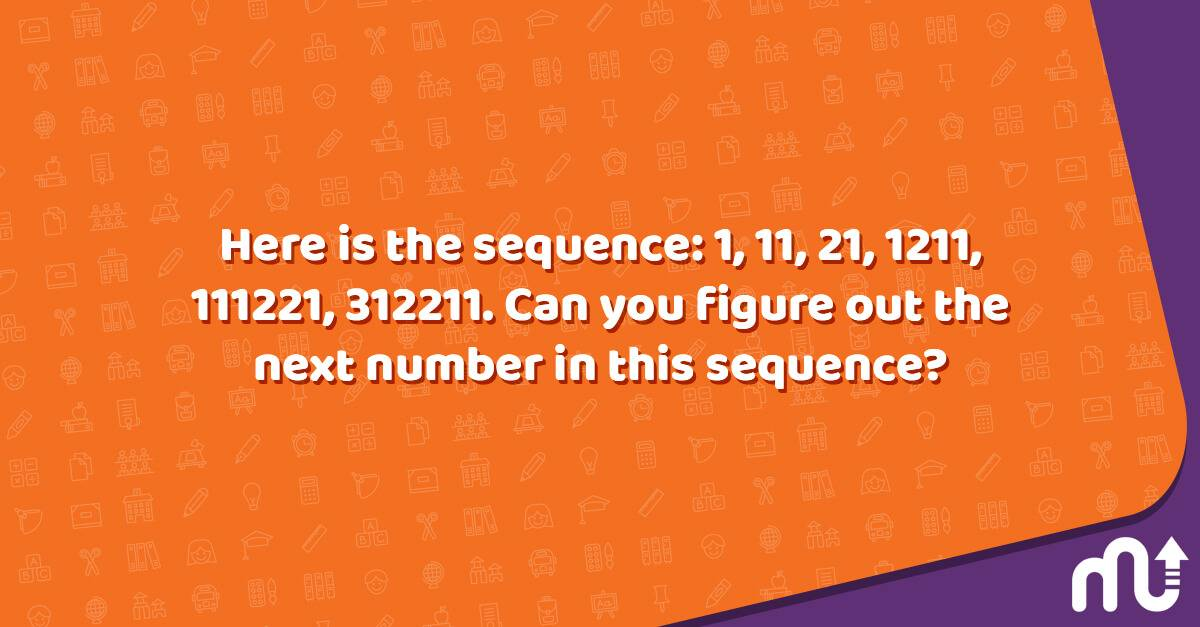Question: Here is the sequence: 1, 11, 21, 1211, 111221, 312211. Can you figure out the next number in this sequence?

Answer: 13112221 (Each of the numbers in the sequence is the description of the previous number. If you start with 1, the 2nd number is 11 (one 1), the third number is 21 (two 1’s), the fourth number is 1211 (one 2, one 1), and so on.
6

### Consecutive Numbers Puzzle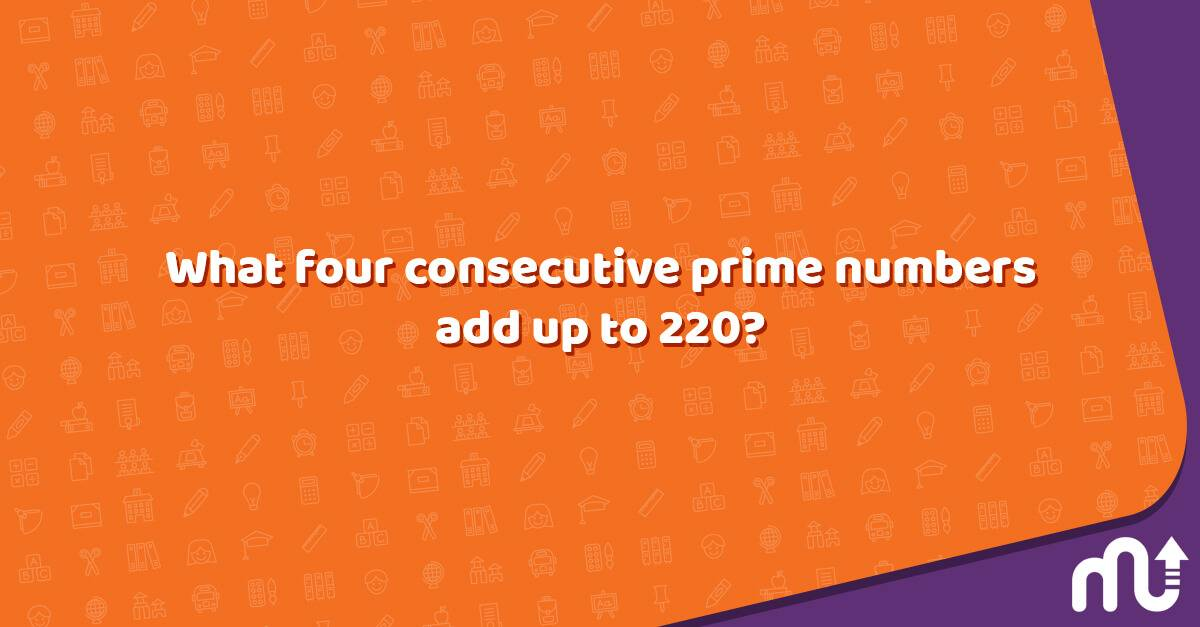Question: What four consecutive prime numbers add up to 220?

Answer: 220 = 47 + 53 + 59 + 61
7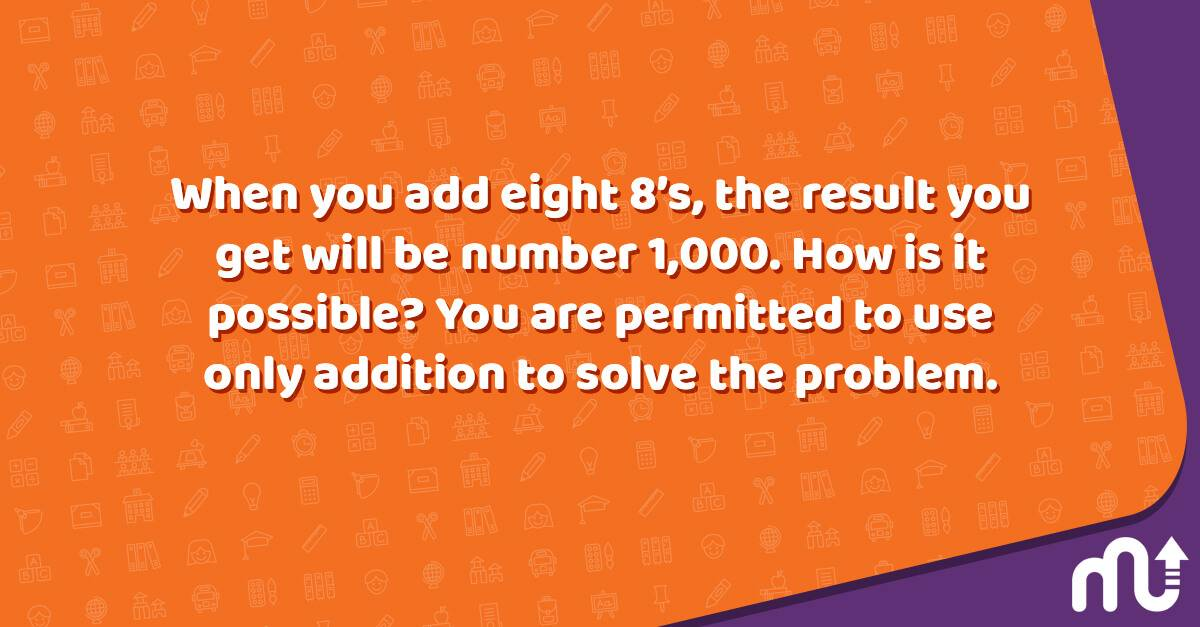Question: When you add eight 8’s, the result you get will be number 1,000. How is it possible? You are permitted to use only addition to solve the problem.

Answer: 888 + 88 + 8 + 8 + 8 = 1,000
8

### Cross Number Puzzle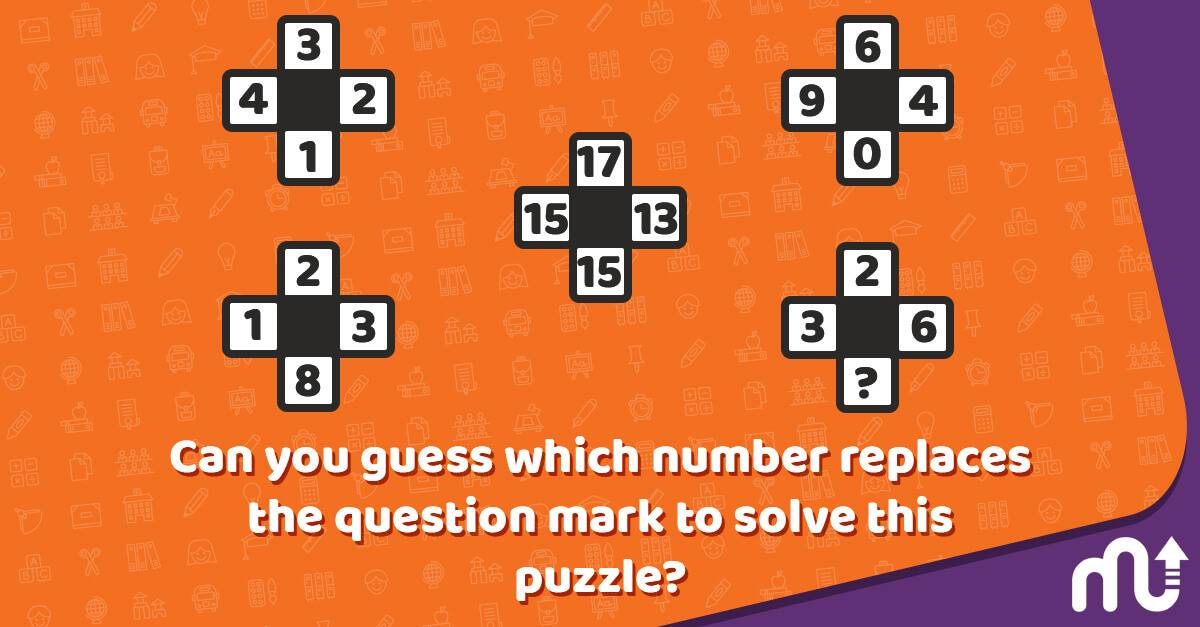Question: Can you guess which number replaces the question mark to solve this puzzle?

Answer: Take values in corresponding positions of the outer 4 shapes, and put the results in the central shape, rotating the position of this result by 1/4 turn each time. So, the answer is 6.
9

### Birthday Puzzle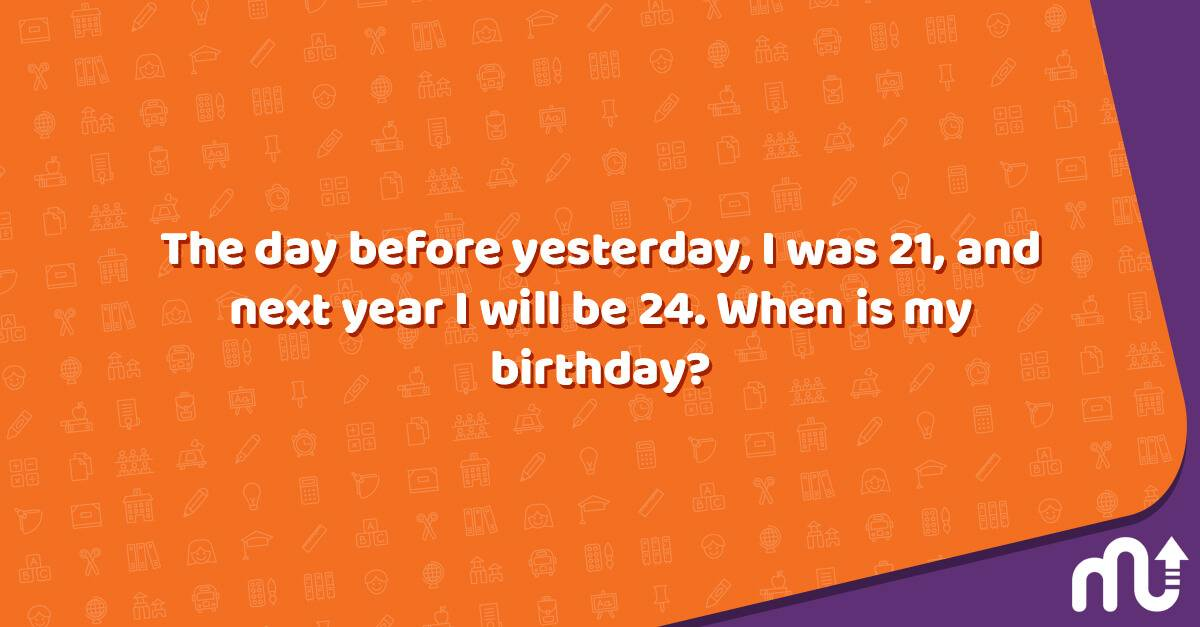Question: The day before yesterday, I was 21, and next year I will be 24. When is my birthday?

Answer: December 31; today is January 1.
10

### Equation Puzzle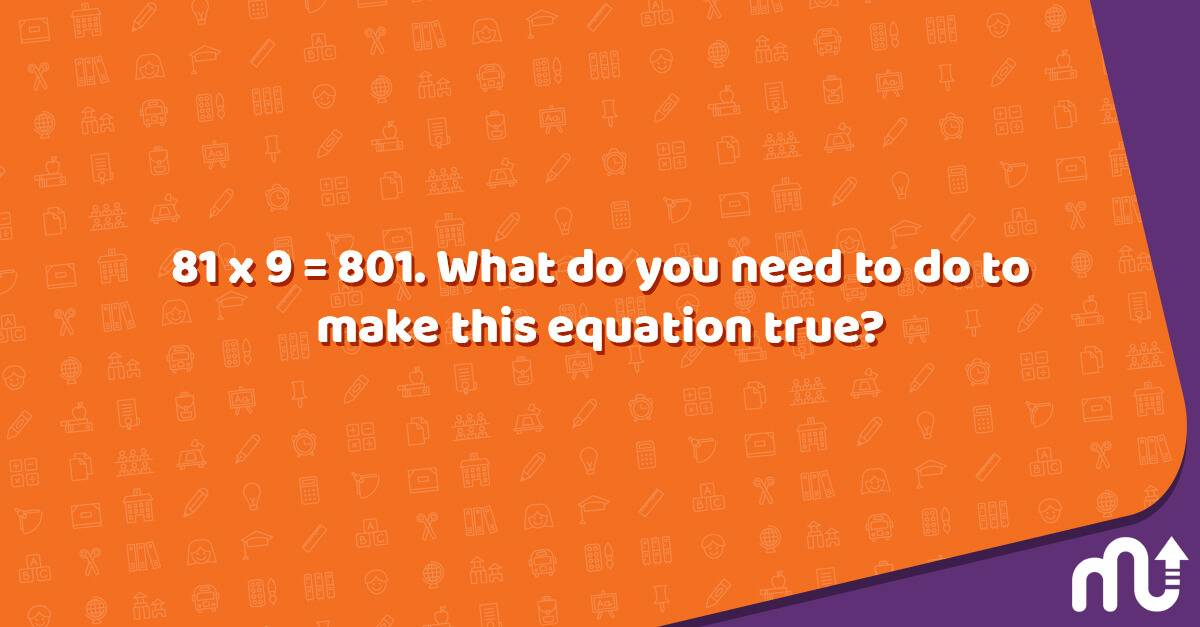Question: 81 x 9 = 801. What do you need to do to make this equation true?

Answer: Turn it upside down. 108 = 6 x 18
11

### Fill-In Number Puzzle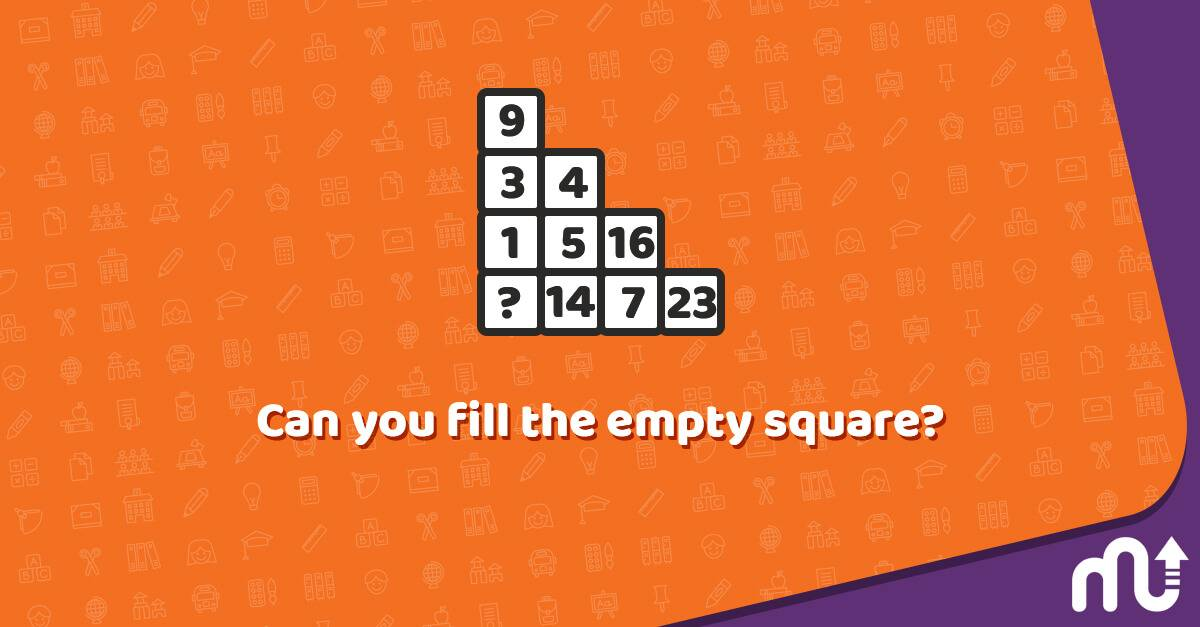Question: Can you fill the empty square?

Answer: Working in columns, the sum of the numbers in each column equals 23. So, the answer is 10.
12

### Clock Puzzle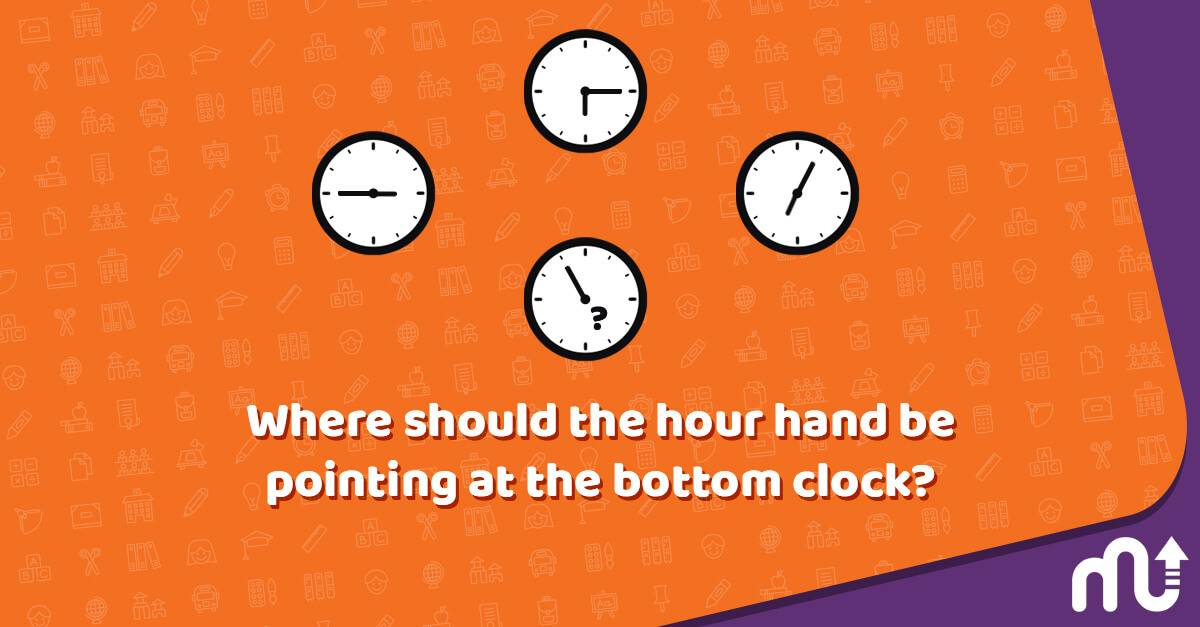Question: Where should the hour hand be pointing at the bottom clock?

Answer: Multiply the hour hand value by 2 and add the minute hand value. This total is always 15, and your hand is pointing to 2.
13

### Diagonal Puzzle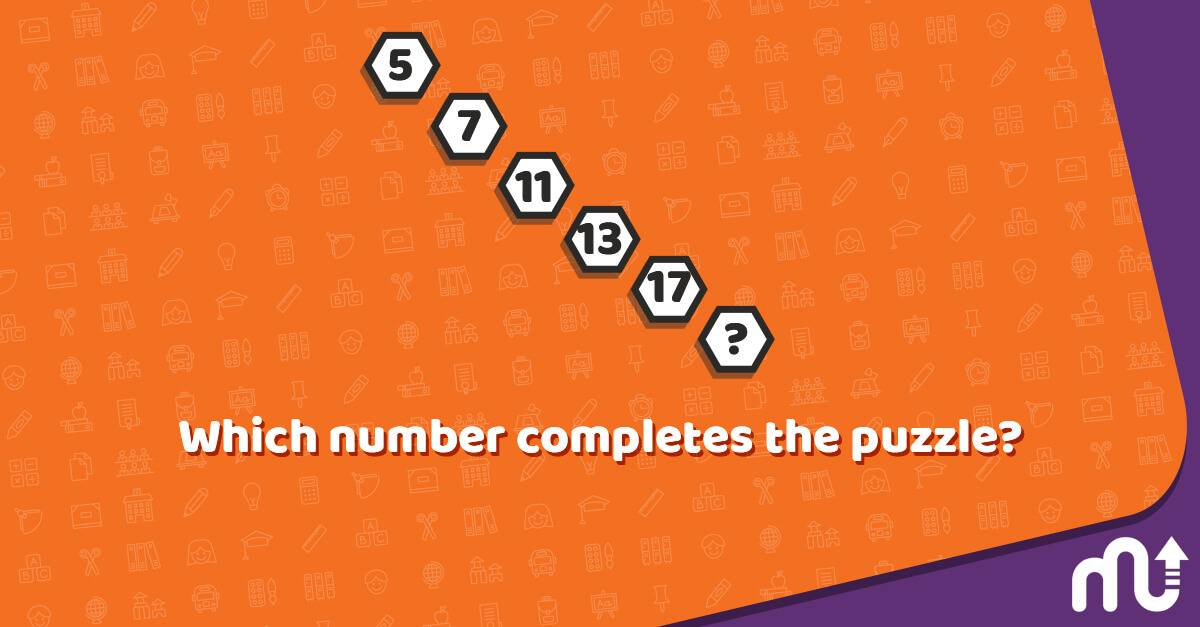Question: Which number completes the puzzle?

Answer: As you move diagonally down, numbers follow the sequence of prime numbers. So, the answer is 19.
14

### Symbol Puzzle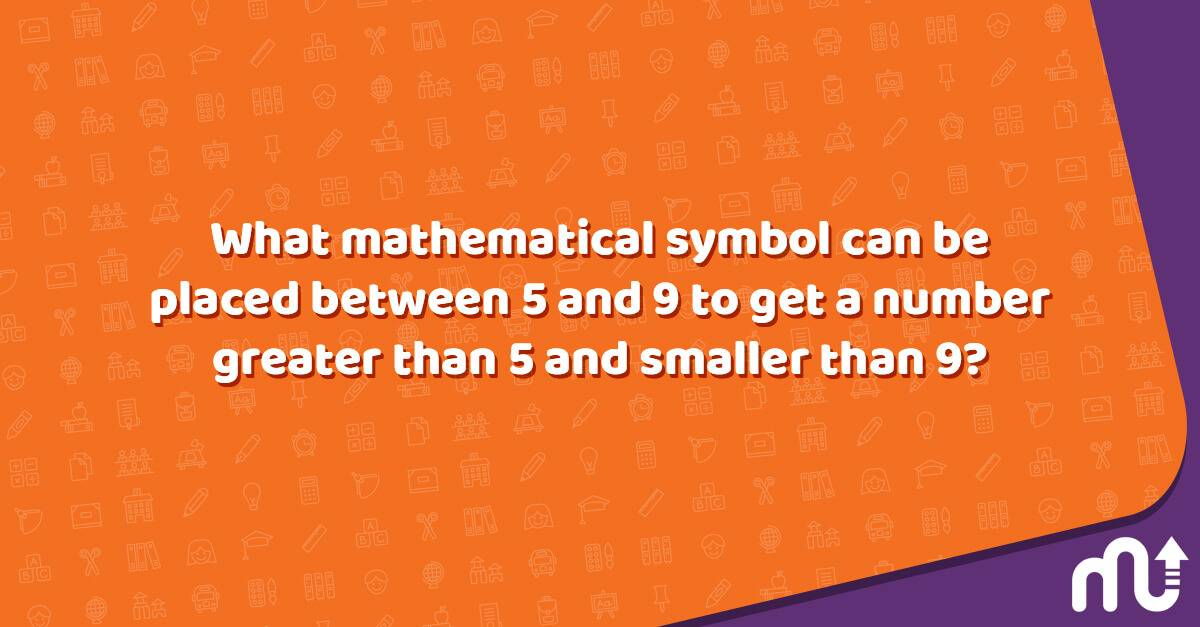Question: What mathematical symbol can be placed between 5 and 9 to get a number greater than 5 and smaller than 9?

Answer: Decimal point to get 5.9
15

### Digit Numbers Puzzle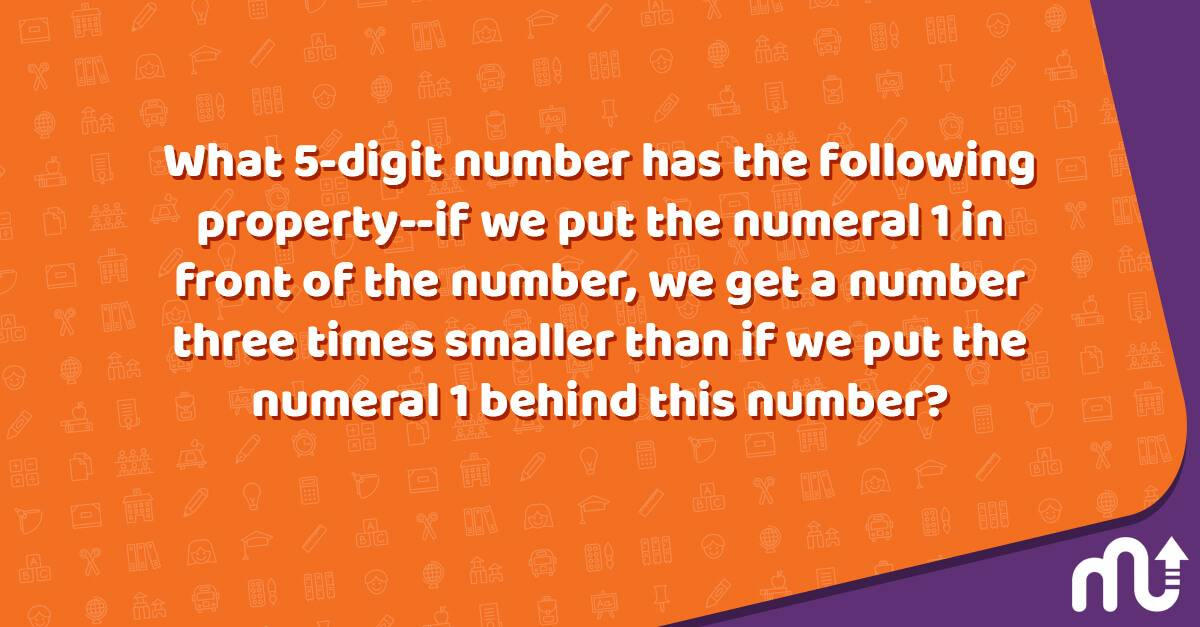Question: What 5-digit number has the following property--if we put the numeral 1 in front of the number, we get a number three times smaller than if we put the numeral 1 behind this number?

Answer: Using an easy equation: 3(x+100000) = 10x+1, we find out that the number is 42857.

Doing puzzles daily is one of the most effective ways to have a healthy brain, and it is good for concentration too. Still, you might need a learning tool supporting your cognitive skills furthermore to stay mentally sharp and take precautions against diseases like dementia.

The multi-awarded app MentalUP is here with its wide range of content specifically designed both for kids and adults. 🏆

From memorization and pattern games to logic exercises and thinking games, you can find all the brain games you need to reinforce your focus, critical thinking, memory, attention, and stress management skills. 🎮

It is time to get started to use MentalUP right now to boost both your and your kid's mental and physical abilities easily! 🎈

## Why Are Number Puzzles Help Kids and Adults?

As we stated before, number puzzles have numerous benefits for both adults and kids. They function like physical exercise for an individual’s brain, so they are essential to having a healthy body and mind. Let's take a closer look at how number puzzles help kids and adults.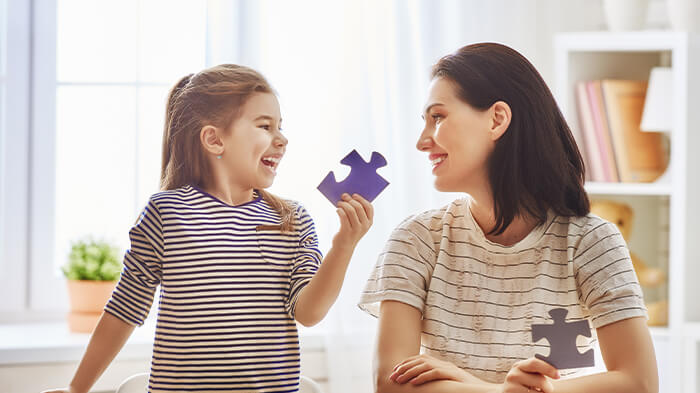• Improve cognitive function, reinforce connections between brain cells, and improve mental processing speed
• Increase the production of dopamine, which is essential to regulating mood, memory, and concentration
• Lower the body pressure and heart rate, which helps individuals relieve stress
• Improve core skills like short-term memory, critical thinking, problem-solving, reasoning, and hand-eye coordination
• Help kids grasp diverse mathematical concepts easier
• Lower the risk of mental diseases like anxiety, dementia, and Parkinson's
• And boost spatial awareness

Throughout this blog, we have explained all the benefits of number puzzles and shared the best number puzzle examples for both kids and adults. If you still have a question left in your mind, here are the most commonly asked questions about number puzzles.

### Where can I find number puzzles?

You can find number puzzle books or resources in your local shops or online. If you want to reach a variety of number puzzles for different ages and skill levels, you can check learning apps like MentalUP. This way, you can find the most appropriate number puzzles for both you and your kid and challenge your minds continuously.

### What are some number puzzles types?

There are a lot of types of number puzzles, like logic number puzzles, triangle number puzzles, grid number puzzles, fill-in number puzzles, or cross number puzzles. Additionally, sudoku and jigsaw puzzle types also fit into this category.

### What are the benefits of number puzzles?

Number puzzles support the overall wellness of people by reinforcing mental health and regulating mood. They are great for exercising the mind, just like memory, mind, concentration, logic, and visual games.

Number puzzles are the most beneficial and enjoyable when supported with other brain exercises and games. If you need a resource to maximize the benefits of number puzzles and improve other cognitive skills along with numeracy and math skills, you can get MentalUP for both you and your kid. 😇

Thanks to its user-friendly interface, the MentalUP app can be used on any PC, tablet, or smartphone. Additionally, it provides you with age and level-appropriate games and activities in each step and enables you to track progress with interactive dashboards. 💪

The certified app MentalUP is here for you with its 150+ educational games and 240+ fitness exercises that are suitable for people of all ages! 📱

YOU MIGHT ALSO LIKE:

#### 68 Best Brain Teaser Puzzles With Answers

HOME PAGE / BLOG / Top 35 Number Puzzles to Keep You Challenged